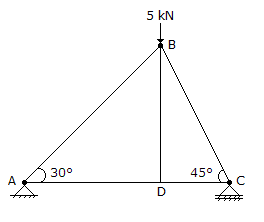# Civil Engineering - UPSC Civil Service Exam Questions

26.

The process by which a mass of saturated soil is caused by external forces to suddenly lose its shear strength and to behave as a fluid is called

 A. piping B. slide C. quick condition D. liquefaction

Explanation:

No answer description available for this question. Let us discuss.

27.

In steady laminar flow of a liquid through a circular pipe of internal diameter D, carrying a constant discharge, the hydraulic gradient is inversely proportional to

 A. D B. D2 C. D4 D. D5

Explanation:

No answer description available for this question. Let us discuss.

28.

A pipe line, 2000 m long, carries water. Velocity of propagation of pressure wave is 1000 m/s. If the valve at the low stream end is instantaneously closed at time, t = 0, negative water hammer pressure at the valve will last for

 A. 0 - 4 S B. 2 - 4 S C. 4 - 8 S D. S - 12 S

Explanation:

No answer description available for this question. Let us discuss.

29.

Consider the following statements related to triaxial test :
1. Failure occurs along pre-determined plane.
2. Intermediate and minor principal stresses are equal.
3. Volume changes can be measured.
4. Field conditions can be simulated.
Of these statements :

 A. 1, 2 and 3 are correct B. 1, 2 and 4 are correct C. 1, 3 and 4 are correct D. 2, 3 and 4 are correct

Explanation:

No answer description available for this question. Let us discuss.

30.

What is the magnitude of the force in the member BD in the figure given above ?A. 5 kN B. 7 kN (App) C. 42kN D. Zero